• +91 9971497814
• info@interviewmaterial.com

# Chapter 6 Lines and Angles Ex-6.1 Interview Questions Answers

### Related Subjects

Question 1 : In the given figure, lines AB and CD intersect at O.If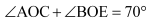and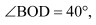find BOE and reflex COE.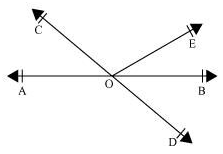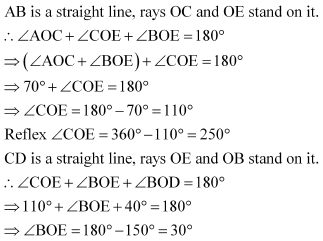Question 2 :

In the given figure, linesXY and MN intersect at O. If POY=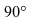and a:b =2 : 3, find c.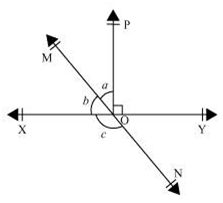Let the common ratiobetween a and b be x.

a =2x, and b =3x

XY is astraight line, rays OM and OP stand on it.

XOM + MOP + POY = 180º

b + a + POY = 180º

3x + 2x +90º = 180º

5x = 90º

x = 18º

a = 2x = 2 × 18 =36º

b = 3x= 3 ×18 = 54º

MN is astraight line. Ray OX stands on it.

b + c = 180º (Linear Pair)

54º + c =180º

c = 180º − 54º = 126º

c =126º

Question 3 :

In the given figure, PQR = PRQ, then prove that PQS = PRT.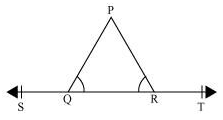In the given figure, ST isa straight line and ray QP stands on it.

PQS + PQR =180º (Linear Pair)

PQR = 180º − PQS (1)

PRT + PRQ = 180º (Linear Pair)

PRQ = 180º − PRT (2)

It isgiven that PQR = PRQ.

Equatingequations (1) and (2), we obtain

180º − PQS = 180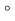− PRT

PQS = PRT

Question 4 : In the given figure, if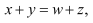then prove that AOB is a line.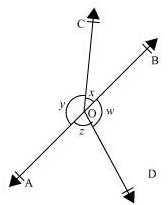It can be observed that,

x + y + z+ w =360º (Complete angle)

It is given that,

x + y = z + w

x + y + x + y =360º

2(x+ y) = 360º

x + y = 180º

Since x and y forma linear pair, AOB is a line.

Question 5 :

In the given figure, POQis a line. Ray OR is perpendicular to line PQ. OS is another ray lying betweenrays OP and OR. Prove that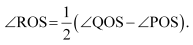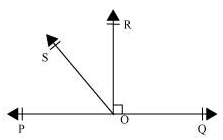It is given that OR PQ

POR = 90º

POS + SOR = 90º

ROS = 90º − POS … (1)

QOR = 90º (As OR PQ)

QOS − ROS = 90º

ROS = QOS − 90º … (2)

On addingequations (1) and (2), we obtain

2 ROS = QOS − POS

ROS =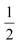(QOS− POS)

Question 6 : It is given that XYZ=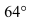and XY is produced topoint P. Draw a figure from the given information. If ray YQ bisects ZYP, find XYQ and reflex QYP.

Answer 6 :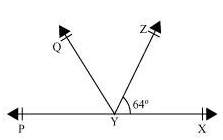It is given that line YQbisects PYZ.

Hence, QYP = ZYQ

It can beobserved that PX is a line. Rays YQ and YZ stand on it.

XYZ + ZYQ + QYP = 180º

64º + 2QYP = 180º

2QYP = 180º − 64º = 116º

QYP = 58º

Also, ZYQ = QYP = 58º

Reflex QYP =360º − 58º = 302º

XYQ = XYZ + ZYQ

=64º + 58º = 122º

Question 7 :

In the given figure, findthe values of x and y and then show that AB || CD.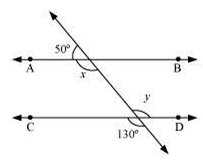It can be observed that,

50º + x = 180º (Linear pair)

x = 130º … (1)

Also, y = 130º (Vertically opposite angles)

As x and y arealternate interior angles for lines AB and CD and also measures of these anglesare equal to each other, therefore, line AB || CD.

Question 8 :

In the given figure, if AB|| CD, CD || EF and y: z = 3: 7, find x.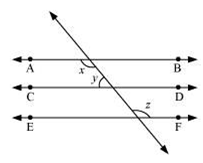It is given that AB || CDand CD || EF

AB || CD || EF (Lines parallel to the sameline are parallel to each other)

It can beobserved that

x = z (Alternateinterior angles) … (1)

It isgiven that yz = 3: 7

Let thecommon ratio between y and z be a.

y =3a and z =7a

Also, x + y =180º (Co-interior angles on the same side of the transversal)

y = 180º [Usingequation (1)]

7a + 3a =180º

10a = 180º

a = 18º

x =7a = 7 × 18º = 126º

Question 9 :

In the given figure, If AB|| CD, EF CD and GED = 126º, find AGE, GEF and FGE.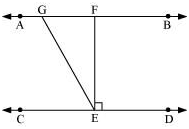It is given that,

AB || CD

EF CD

GED = 126º

GEF + FED =126º

GEF + 90º = 126º

GEF = 36º

AGE and GED are alternate interior angles.

AGE = GED =126º

However, AGE + FGE = 180º (Linear pair)

126º + FGE = 180º

FGE = 180º − 126º = 54º

AGE = 126º, GEF =36º, FGE = 54º

Question 10 :

In the given figure, if PQ|| ST, PQR =110º and RST =130º, find QRS.

[Hint: Draw a line parallel to STthrough point R.]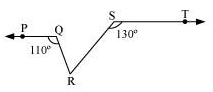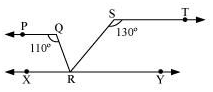Let us draw a line XYparallel to ST and passing through point R.

PQR + QRX = 180º (Co-interior angles on the same side of transversal QR)

110º + QRX = 180º

QRX = 70º

Also,

RST + SRY = 180º (Co-interior angles on the same side of transversal SR)

130º + SRY = 180º

SRY = 50º

XY is astraight line. RQ and RS stand on it.

QRX + QRS + SRY = 180º

70º + QRS + 50º = 180º

QRS = 180º − 120º = 60º

Todays Deals### Chapter 6 Lines and Angles Ex-6.1 Contributorskrishan

Name:
Email:

# Latest News# 9000 interview questions in different categories